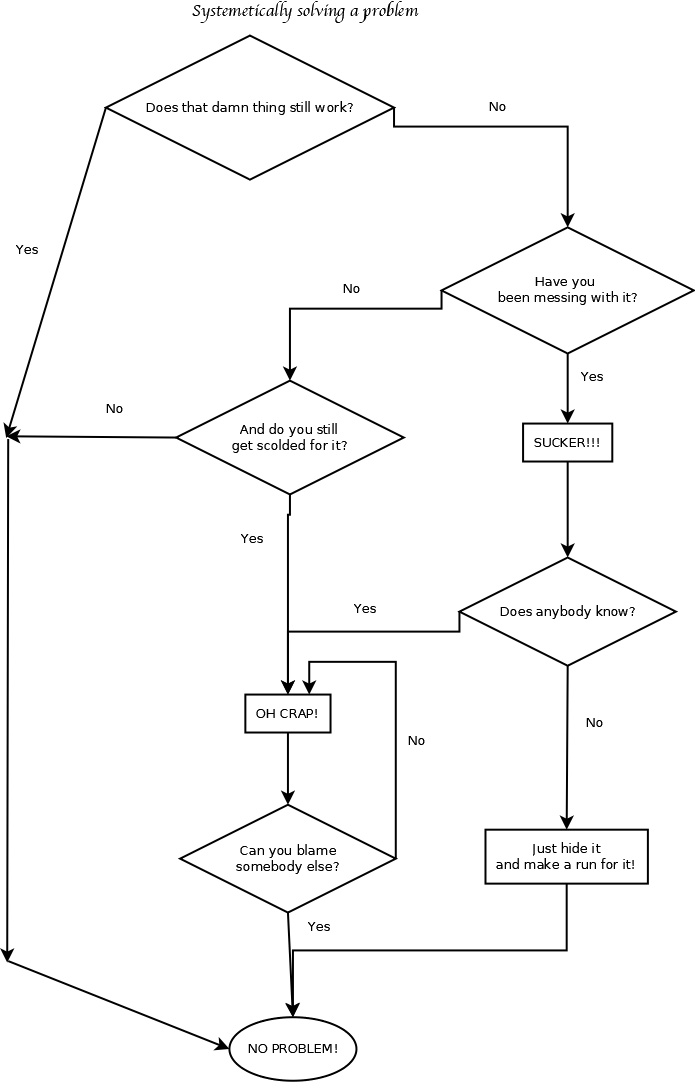-=+=- -=+=- -=+=- -=+=- -=+=- -=+=- -=+=- -=+=- -=+=- -=+=- -=+=- -=+=- -=+=- -=+=- -=+=- -=+=- -=+=- -=+=- -=+=- -=+=- -=+=- -=+=- -=+=- -=+=- -=+=- -=+=- -=+=- -=+=- -=+=- -=+=- (c) WidthPadding Industries 1987 0|685|0 -=+=- -=+=- -=+=- -=+=- -=+=- -=+=- -=+=- -=+=- -=+=- -=+=- -=+=- -=+=- -=+=- -=+=- -=+=- -=+=- -=+=- -=+=- -=+=- -=+=- -=+=- -=+=- -=+=- -=+=- -=+=- -=+=- -=+=- -=+=- -=+=- -=+=-
Socoder -> Off Topic -> Systematic solving a problem

Posted : Monday, 02 December 2019, 09:55
Tricky### Systematic solving a problemI shall be honest, I drew this version myself, but I was not the one who made up the original joke which I received in Dutch and I merely redrew and translated it. However funny as this may be this joke does show a thing or two about how to set up a nice diagram in how to solve problems and issues which can also help a lot when running a game project or a coding project in general. But these diagrams are not limited to coding alone.

I used the open-source program "Dia" to draw this.Posted : Wednesday, 04 December 2019, 17:16
JayenkaiThis is very informative. That bottom right thing seems to have fixed a ton of my issues.

-=-=-